# Mathematical Modelling of Control System | Mechanical Electrical

## Mathematical Modelling of Control System

There are various types of physical systems, namely we have:

1. Mechanical systems
2. Electrical systems
3. Electronic systems
4. Thermal systems
5. Hydraulic systems
6. Chemical systems

First off we need to understand – why do we need to model these systems in the first place? Mathematical modeling of a control system is the process of drawing the block diagrams for these types of systems in order to determine their performance and transfer functions.

Now let us describe the mechanical and electrical type of systems in detail. We will derive analogies between mechanical and electrical system only which are most important in understanding the theory of control system.

### Mathematical Modelling of Mechanical Systems

We have two types of mechanical systems. The mechanical system may be a linear mechanical system or it may be a rotational mechanical type of system.
In a linear mechanical type of systems, we have three variables:

1. Force, represented by ‘F’
2. Velocity, represented by ‘V’
3. Linear displacement, represented by ‘X’

And also we have three parameters:

1. Mass, represented by ‘M’
2. The coefficient of viscous friction, represented by ‘B’
3. The spring constant, represented by ‘K’

In a rotational mechanical type of systems we have three variables:

1. Torque, represented by ‘T’
2. Angular velocity, represented by ‘ω’
3. Angular displacement, represented by ‘θ’

And also we have two parameters :

1. Moment of inertia, represented by ‘J’
2. The coefficient of viscous friction, represented by ‘B’

Now let us consider the linear displacement mechanical system which is shown below-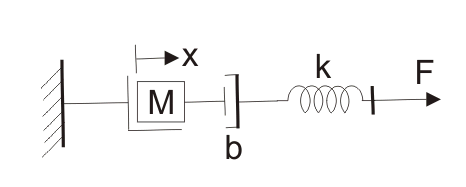We have already marked various variables in the diagram itself. We have x is the displacement as shown in the diagram. From the Newton’s second law of motion, we can write force as-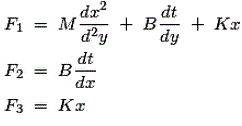From the diagram below we can see that: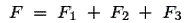On substituting the values of F1, F2 and F3 in the above equation and taking the Laplace transform we have the transfer function as,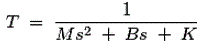This equation is the mathematical modeling of a mechanical control system.

### Mathematical Modelling of Electrical System

In an electrical type of system we have three variables –

1. Voltage which is represented by ‘V’.
2. Current which is represented by ‘I’.
3. Charge which is represented by ‘Q’.

And also we have three parameters which are active and passive components:

1. Resistance which is represented by ‘R’.
2. Capacitance which is represented by ‘C’.
3. Inductance which is represented by ‘L’.

Now we are in condition to derive analogy between electrical and mechanical types of systems. There are two types of analogies and they are written below:
Force Voltage Analogy : In order to understand this type of analogy, let us consider a circuit which consists of series combination of resistor, inductor and capacitor.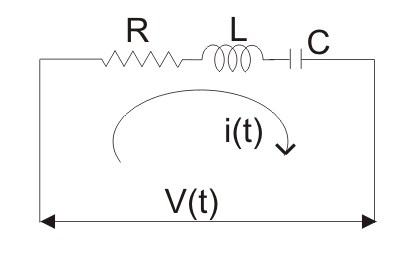A voltage V is connected in series with these elements as shown in the circuit diagram. Now from the circuit diagram and with the help of KVL equation we write the expression for voltage in terms of charge, resistance, capacitor and inductor as,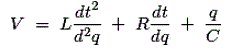Now comparing the above with that we have derived for the mechanical system we find that-

1. Mass (M) is analogous to inductance (L).
2. Force is analogous to voltage V.
3. Displacement (x) is analogous to charge (Q).
4. Coefficient of friction (B) is analogous to resistance R and
5. Spring constant is analogous to inverse of the capacitor (C).

This analogy is known as force voltage analogy.
Force Current Analogy : In order to understand this type of analogy, let us consider a circuit which consists of parallel combination of resistor, inductor and capacitor.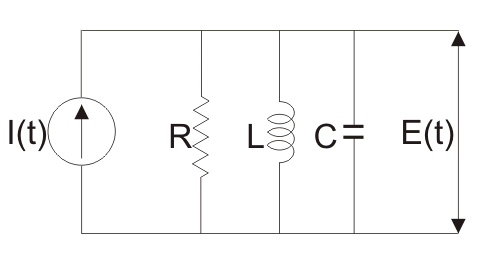A voltage E is connected in parallel with these elements as shown in the circuit diagram. Now from the circuit diagram and with the help of KCL equation we write the expression for current in terms of flux, resistance, capacitor and inductor as,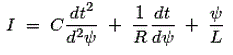Now comparing the above with that we have derived for the mechanical system we find that,

1. Mass (M) is analogous to Capacitor (C).
2. Force is analogous to current I.
3. Displacement (x) is analogous to flux (ψ).
4. Coefficient of friction (B) is analogous to resistance 1/ R and
5. Spring constant K is analogous to inverse of the inductor (L).

This analogy is known as force current analogy.
Now let us consider the rotational mechanical type of system which is shown below we have already marked various variables in the diagram itself. We have θ is the angular displacement as shown in the diagram. From the mechanical system, we can write equation for torque (which is analogous to force) as torque as,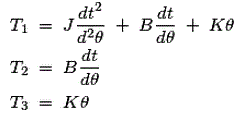From the diagram we can see that the,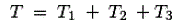On substituting the values of T1, T2 and T3 in the above equation and taking the Laplace transform we have the transfer function as,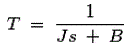This equation is mathematical modelling of electrical control system.

Want To Learn Faster? 🎓
Get electrical articles delivered to your inbox every week.
No credit card required—it’s 100% free.